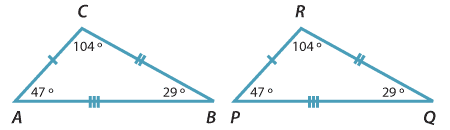## Congruent triangles

When geometric figures are made up entirely of intervals, rather than curves, arguments about congruence can usually be reduced to arguments about congruent triangles. This is one reason why so much attention is given to congruent triangles.

Here are two congruent triangles. The triangle $$PQR$$ on the right has been formed by a translation of the triangle $$ABC$$ on the left.The vertices and sides of triangle $$ABC$$ are matched by the congruence with the vertices and sides of triangle $$PQR$$ as follows:

 $$A\leftrightarrow P$$ $$B\leftrightarrow Q$$ $$C\leftrightarrow R$$ $$BC\leftrightarrow QR$$ $$CA\leftrightarrow RP$$ $$AB\leftrightarrow PQ$$

All this can be expressed extremely concisely by using the symbol $$\equiv$$ for 'is congruent to', and by observing the convention that the two triangles are named with the vertices in matching order:

$$\triangle ABC \equiv \triangle PQR$$